# The Building Blocks of Matter AtomsPage 4

#### WATCH ALL SLIDES

The force that holds the electrons around the nucleus

-

+

+

+

-

-

Notice how the particles with the same charge move apart and the particles with different charges move together.

Why are neutrons not pictured above?

Slide 24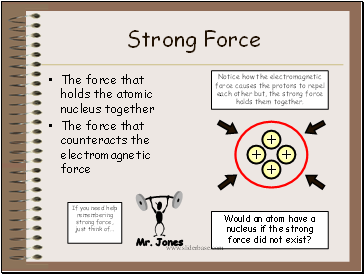## Strong Force

The force that holds the atomic nucleus together

The force that counteracts the electromagnetic force

Mr. Jones

If you need help remembering strong force, just think of…

+

+

+

+

Notice how the electromagnetic force causes the protons to repel each other but, the strong force holds them together.

Would an atom have a nucleus if the strong force did not exist?

Slide 25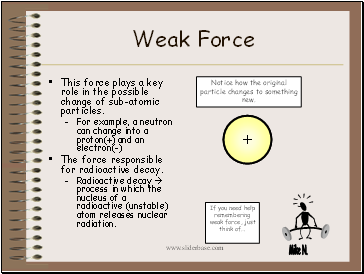-

n

## Weak Force

This force plays a key role in the possible change of sub-atomic particles.

For example, a neutron can change into a proton(+) and an electron(-)

The force responsible for radioactive decay.

Mike N.

+

If you need help remembering weak force, just think of…

Notice how the original particle changes to something new.

Slide 26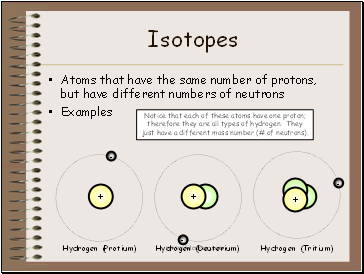## Isotopes

Atoms that have the same number of protons, but have different numbers of neutrons

Examples

+

-

+

-

+

-

Hydrogen (Protium)

Hydrogen (Deuterium)

Hydrogen (Tritium)

Notice that each of these atoms have one proton; therefore they are all types of hydrogen. They just have a different mass number (# of neutrons).

Slide 27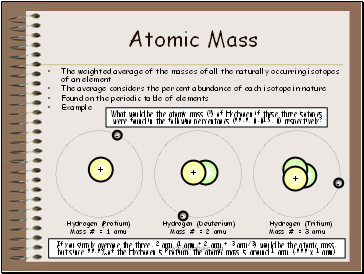## Atomic Mass

The weighted average of the masses of all the naturally occurring isotopes of an element

The average considers the percent abundance of each isotope in nature

Found on the periodic table of elements

Example

+

-

+

-

+

-

Hydrogen (Protium)

Mass # = 1 amu

Hydrogen (Deuterium)

Mass # = 2 amu

Hydrogen (Tritium)

Mass # = 3 amu

If you simply average the three, 2 amu (1 amu + 2 amu + 3 amu/3) would be the atomic mass, but since 99.9% of the Hydrogen is Protium, the atomic mass is around 1 amu (.999 x 1 amu)

What would be the atomic mass (≈) of Hydrogen if these three isotopes

were found in the following percentages (99.9, 0.015, 0) respectively?

Slide 28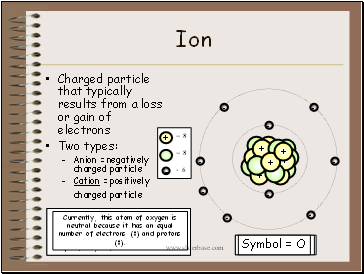## Ion

Symbol = O2+

Charged particle that typically results from a loss or gain of electrons

Two types:

Anion = negatively charged particle

Cation = positively charged particle

Go to page:
1  2  3  4  5